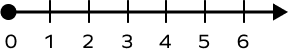# Ray in Math – Definition, Examples, Practice Problems, FAQs,

Home » Math-Vocabluary » Ray in Math – Definition, Examples, Practice Problems, FAQs,

We come across various angles in our everyday life, such as the hands of a clock, a slice of pizza, and an arrowhead showing direction, to name a few. To structure an angle, we need to know what a ray is in math. Rays help us form different angles depending on how we arrange them.

Today, we shall find out what a ray is in math. Come, let’s begin!

## What Is a Ray in Math?

The definition of ray in math is that it is a part of a line that has a fixed starting point but no endpoint. It can extend infinitely in one direction. Since a ray has no end point, we can’t measure its length.

Fun Facts: The sun rays are an example of a ray. The sun is the starting point or the point of origin, and its rays of light extend indefinitely in our solar system.

## Naming a Ray in Math

On its way to infinity, a ray may pass through more than one point. A ray is named using its initial point and any other point through which it passes. So, the first letter of a ray’s name indicates its starting point.

When naming a ray, it is denoted by drawing a small ray on top of the name of the ray.

The figure below represents a ray PQ. Here, the starting point of ray PQ is P, and on its way to infinity, it passes through point Q.

Now consider the diagram below. It has a ray that passes through two points on its endless journey. The starting point of this ray is D. You can name it ray DE or ray DF.

Fun Fact: The point from where a ray starts its journey towards infinity is called its endpoint!

## Rays and Angles

In geometry, when two rays share a common endpoint, they form an angle.

Here, in the below figure, each of the angles is made up of two rays. The vertex of the angles is the starting point of the rays. It is the vertex that gives us the measure of an angle. The rays from the arms of the angles.

Angles are measured in degrees (°).

In the below figure, the angle ABC is formed by the rays BA and BC.

## Points to Remember about Rays

• A ray is a geometric figure that has no height or width. It only has an indefinite length.
• The name of a ray must always include its origin point.
• We need two rays to form an angle.

## Conclusion

Having a clear idea about a ray in math is important. It helps us understand the concept of angles and multiple angles enable us to form a polygon

## Solved Examples On Ray in Math

Example 1: What is the endpoint of ray GF in the given figure below?

Solution: In the given figure, the endpoint of ray GF is G. Since the origin point of a ray is called its endpoint, here G is the endpoint.

Example 2: Which rays are opposite to each other in the figure below?

Solution: Ray GH and ray GC are opposite rays in the given figure. These rays start from point G and proceed in the opposite direction to form a straight angle.

Example 3: Write the names of any five rays as seen in the given figure.

Solution: Ray OC, ray OA, ray OG, ray CA, and ray GS are five rays seen in the given figure. Here, ray OC, ray OA, and ray OG originate from the point O. Ray CA has C as the endpoint, and ray GS has G as the endpoint.

## Practice Problems On Ray in Math

1

### Which of the given figures has an indefinite length?

A line segment
A ray
A triangle
A circle
CorrectIncorrect
Since a ray extends endlessly in one direction, it has no definite length. But all the other given figures have definite length.
2

### If a line is divided into two parts, what will be formed?

One line segment and one ray
Two line segments
Two rays extending in the same direction
Two opposite rays
CorrectIncorrect
Correct answer is: Two opposite rays
When a line is divided into two parts, it will form two rays that extend in the opposite direction.
3

### How many rays can you draw from a given point?

One
Four
Three
Infinite
CorrectIncorrect
When you are given a point, you can draw an endless number of rays from that point in every direction.
4

### Consider the number line representing whole numbers. Which one of these statements is true?It is a ray.
It is a line.
It is a line segment.
None of the above.
CorrectIncorrect
Correct answer is: It is a ray.
The number line representing whole numbers starts from a fixed point, 0. It then extends endlessly through 1 as there is no end to whole numbers.

## Frequently Asked Questions On Ray in Math

A ray has a fixed starting point and extends endlessly in another direction. But a line has no fixed starting point and extends endlessly in both directions.

Yes, we can extend a ray infinitely in one direction. A ray starts from a fixed point and so, it can’t be extended infinitely in both directions.

A ray has zero thickness. It is a one-dimensional figure that has an indefinite length.

The symbol of a ray is a small arrow (->) that is placed above the name of the ray.